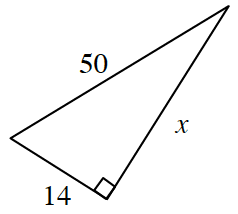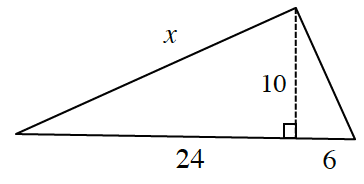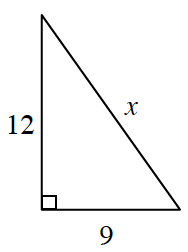### Home > INT2 > Chapter 6 > Lesson 6.1.4 > Problem6-43

6-43.

Use what you know about Pythagorean Triples to determine the measure of the third side.

Some common Pythagorean Triples are $(3,4,5)$, $(5,12,13)$, $(8,15,17)$, and $(7, 24, 25)$.

1.Find a common factor between each of the given side lengths.

1.$5$, $12$, $13$ pattern  $x = 26$

1.Refer to the hint in part (a).